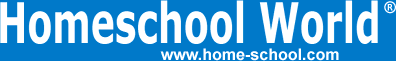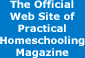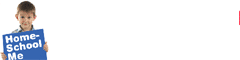Home Curriculum Catalog Articles Contests Events Groups Forum News ContactGetting Started Preschool/K Elementary Middle School High School College & Career Prep Special Needs Group Activities Research FAQs# Calculus: The Bridge to College Math and Science

By Bill Pride
Printed in Practical Homeschooling #69, 2006.Taming the 10-ton gorilla of math coursesCalculus is definitely considered the 10-ton gorilla of math courses for a high school student. But once you get into it, calculus turns out to be less like King Kong and more like Cheetah.

Why Take Calculus?

First, calculus looks impressive on your transcript. Colleges know that any high-school student who can do calculus is a student who can do well in college.

Second, "engineering" style college physics requires calculus. If you learn your calculus before you reach your freshman year, think of the advantage you will have over your comrades who are taking Calculus I concurrently.

Third, you may be able to skip a semester of calculus and substitute something more interesting in your first semester.

What is Calculus?

Anyone who has braved algebra and geometry and has fought his way through advanced algebra should have no problem conquering calculus.

Calculus continues the study of functions. A function is like a computing machine. You put in a number and you get a number back out. If you put the same number in, you get the same number out every time. For example, look at f(x) = x2. Every time you put in 2 you get out 4. Every time you put in 3 you get out 9. What makes f(x) a function is that each different value for x produces only one value of f(x).

Calculus does basically four things with functions.

• It finds their limit at various values of x
• It determines if they arecontinuous or not
• It differentiates them
• It integrates them

The limit of a function tells you what f(x) does as x sneaks up on some value. The question goes like this, as x goes from 2 toward 3, what does f(x) do? If, as x gets closer and closer to 3, f(x) gets closer and closer to some particular number, such that you can get f(x) as close as you want to that number by getting x close enough to 3, then that number is the limit of f(x) as x goes to 3. If f(x) goes to the same number when you sneak up on 3 from the high side (when you're going from 4 to 3), then you say f(x) has a limit at 3. Looking at f(x) = x2 again: as x gets closer and closer to 3 whether you approach from the 4 side or the 2 side, x2 gets closer and closer to 9. So the limit of x2 when x approaches 3 is 9. You can get x2 as close as you want to 9 by getting x close to 3. Let's say you want x2 to be within .01 of 9. You can do that by making x be less than .001 away from 3. (Try squaring 2.999 and 3.001 and see if it works.)

Continuous means that the limit of f(x) as x approaches some number, call it x0, is f(x0). So, since the limit of x2 as x goes to 3 is 9 and f(3) = 32 = 9, we say f(x) = x2 is continuous at 3. In fact, x2 is continuous at every value for x you could think of, so we say f(x) = x2 is continuous. Calculus wants functions to be continuous, because if a function is continuous you can do the next thing to it which is to differentiate it.

What's a differential? If f(x) tells you where you are, the differential tells you whether f(x) is increasing or decreasing and how fast. If you drew a graph of f(x) and at some point on the graph you drew a line tangent to the graph, the slope of that line would be the differential. If you were travelling and f(t) (t = time) told you how far you were from home, the differential of f(t) would tell you how fast you were going. This kind of thing is very useful in physics.

The fourth thing you learn in calculus - the integral - is the inverse of the differential. If you integrate the differential of a function, you get the original function back again, more or less. The function you get might differ from the original function by adding a constant to it.

Integrals add things. For example, if you have a function and for some reason you want to find the area between the graph of the function and the x-axis (the line where f(x) is equal to zero) over some range of values for x, you would take the integral of the function, evaluate it at the high end of the range and subtract its value at the low end of the range, and that would be the area. What you are doing, in theory, is dividing the area into a bunch of very thin vertical rectangles and adding up their areas.

Dividing things up into tiny pieces and adding up the contribution of each of the tiny pieces to some total effect is a very useful thing to be able to do and that is what integration is all about.

In calculus you learn to do the four things above to a wide variety of different kinds of functions using a dozen different methods and that's about it. And since you learn to do it systematically in small steps over a whole school year it really isn't that difficult.

How do I Teach Calculus?

Like any homeschool subject, the cost of teaching calculus goes down the more work the teacher does. Theoretically, you could teach calculus to one student with two dozen legal pads and a dozen pencils if you knew the subject well enough.

The next step up would be a calculus text book. Saxon Math is the calculus textbook of choice for many homeschoolers at about \$75 for the whole package, including tests, answer key, and solutions manual. If you go to Amazon you can find lots of other calculus textbooks from \$100-\$160.

A new contender that just arrived on our doorstep this week is the Life of Fred hardbound calculus text from Polka Dot Publishing. Costing even less than Saxon, at \$39, it takes a less dignified and more entertaining (but still systematic) approach to calculus.

Calculus tutoring software exists, such as GraspMath Calculus (\$199.95). D.I.V.E. has a CD full of video solutions worked on a whiteboard for all the problems in the Saxon Calculus course for \$50. The CD together with the Saxon text costs \$135.

At the high end for at-home, do-it-yourself learning is Chalk Dust's calculus course. The 4 DVDs cost \$75 apiece. The complete course with DVDs, textbooks, and solutions manual, plus free consulting time via telephone or email with the on-tape instructor, costs \$409.

You can get at-home distance learning Advanced Placement (AP) Calculus from apexlearning.com for about \$700. If you just want to take advantage of APEX's AP exam review for calculus, that will run you \$49. The exam review is now sold through the Princeton Review site at www.princetonreview.com.

If you are willing to drive a little, you can take calculus for dual credit at a community college or a local 4-year college or university. Our community college charges \$78/credit hour for area residents. Calculus is 5 credit hours, so this comes to \$390 per semester. After buying the textbook and calculator, you probably will spend \$600 or so. A Christian college near us offers dual credit courses for \$90/credit hour, but since their Calculus I is only four credit hours, this comes to \$360 per semester plus textbook and calculator. The advantage of dual credit is that you get both high-school and college credit for the course without having to take the AP exam (which costs another \$80).

To AP or not to AP?

There are two reasons to take calculus in high school - either you just want to familiarize yourself with the subject for when you take calculus in college, or you want to place out of a semester of college calculus. Any calculus course will do for the first goal. In order to place out of a semester of calculus assuming your intended college offers this option, you need to prepare for and successfully take one of the Calculus AP exams, or a dual credit course at a regionally accredited college.

AB or BC?

There are two Calculus AP exams: the AB exam and the BC exam, with the second being the more difficult. Which one you prepare for depends on the college you wish to attend. At University of Missouri-St. Louis, for example, a 3 on the AB exam will earn 5 calculus credits and a 4 on the BC exam will earn 10 calculus credits. MIT requires a 4 or 5 on the BC exam to get credit for the entire Calculus I course. A 4 or 5 on the AB only entitles you to enter an accelerated Calculus I/Calculus II sequence. Check the requirements for the schools you're aiming for and take the test you need for those schools.

Calculus can be a real plus in your high-school program. It will help you stand out from the crowd when it's time to apply to college, and likewise improve your scholarship chances. With a little diligence and step-by-step study you can integrate calculus successfully into your high school curriculum.

Where to Find It

APEX Learning, WA. (800) 453-1454. www.apexlearning.com

Chalk Dust, TX. (800) 588-7564. www.chalkdust.com

D.I.V.E. into Math and Science. (936) 372-9216. www.diveintomath.com

GraspMath, LA. (877) 843-0277. www.sieducation.com

Polka Dot Publishers, NV. (775) 852-2690. www.polkadotpublishing.com

Saxon Publishers/Harcourt Achieve, OK. (800) 284-7019. www.saxonpub.comTerms of Use   Privacy Policy Copyright ©1993-2023 Home Life, Inc.